# Operations with sets

The set B - A has twice as fewer elements than the set A - B and four times fewer elements than the set A ∩ B. How many times more elements does the set A have than the set B?

k =  1.2

### Step-by-step explanation: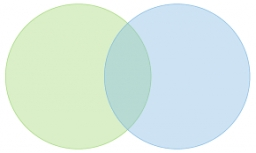Did you find an error or inaccuracy? Feel free to write us. Thank you!## Related math problems and questions:

• Subtracting sets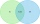For two sets K, L is true: K has 30 elements, L has 27 elements and the set L - K has 22 elements. How many elements does the set K - L have?
• Two sets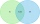Suppose Set B contains 69 elements and the total number elements in either Set A or Set B is 124. If the Sets A and B have 29 elements in common, how many elements are contained in set A?
• They boughtThey bought the students a set of notebooks for 65 euros. The first set cost € 2 and the second € 3. There were 5 times as many two-euro sets as three-set ones. How many sets of workbooks are there?
• DisjointHow many elements have union and intersection of two disjoint sets when the first have 1 and secodn 8 elements.
• PhotosThree friends Mirka, Lucka, and Hanka, collect photos. If Mirka had 3 more photos than she has, she would have as many as Hanka. If Lucka had 5 photos less than she has, she would be like Hanka. How many photographs does each have if they have 431 togethe
• Balls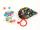Michal said to Martin: give me one ball, and I'll have twice as you. Martin said: give me 4, and we will have equally. How many balls each have?
• At theAt the presentation of the travelers came three times as many men than women. When eight men left with their partners, there were five times more men than women at the presentation. How many were men and women originally?
• Aircraft modelsHow many car models did the collector own after the collector's market? The collector of car and aircraft models owned 3 times more car models than aircraft. He sold 7 car models and 9 aircraft models on the stock exchange and bought 12 car models and 4 a
• DollarsMama split 760 dollars Jane, Dane and Eva as follows: Jane got three times more than Dane and Dane and got 40 more than Eva. How much does get each of them?
• Two unknown numbersOne number is 2 less than a second number. Twice the second number is 14 less than 5 times the first. Find the two numbers.
• Count of roots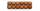How many solutions has equation x. y = 7757 with two unknowns on the set of natural numbers?
• Peter and PaulPeter and Paul together have 26 years. Four years ago, Paul was twice older than Peter. How much is Paul and how much Peter?
• The sum 8The sum of two numbers is 21. If three times the smaller numbers is two less than twice the larger number, find the two numbers.On the meadow grazing horses, cows, and sheep, together with less than 200. If cows were 45 times more, horses 60 times more, and sheep 35 times more than there are now, their numbers would equally. How many horses, cows, and sheep are on the meadow togetAndrew, Lenka and Rasto have together 232 pears. Lenka has 28 more than Rasto and Rasto pears have 96 more than Andrew. Determine how much each of them has pears.Peter has 2x more money than Michael. But if Peter gave Michael € 16 Michael would have 3.5 times more money than Peter. Determine how much money was originally Peter and how much Michael.6 years ago, Marcela's mother was two times older than her and two times younger than her father. When Marcela is 36, she will be twice as young as her father. How old are Marcela, her father, and her mother now?